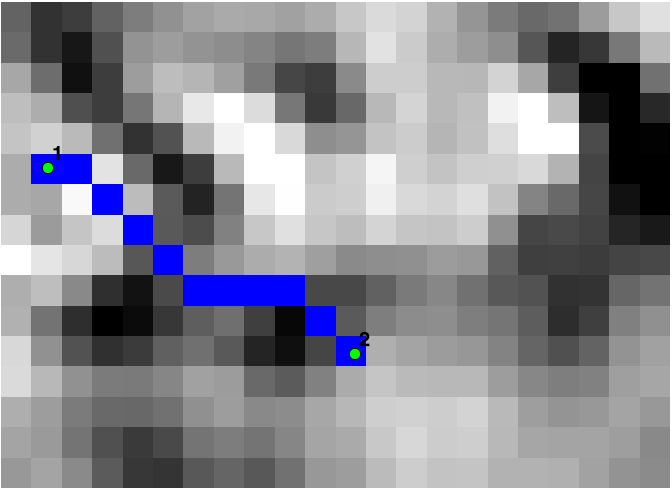# Least Cost Path or How to Avoid Volcanoes with GrassGIS

Recently (ok, I have been working on-and-off on this for longer than I’d like to admit) we needed to solve a least cost path problem. I poked around and looked at a few solutions.One was in Jared Erikson’s Python GDAL/OGR Cookbook which was fun to play with. This is a fun solution as it’s pretty low level, so you can see all the parts and pieces. Check it out: it may be a useful think if you really need a python only solution.

Another solution is to use GRASS GIS. The trick is to use calculate the cost surface between two points, and then proverbially let the marble roll down that cost surface.

First the problem — what the most efficient way (ignoring roads, trails and other affordances) to get from one side of Volcan Muhabura (on the border of Rwanda and Uganda) to the other? In a few weeks I hope to hike that mountain, assuming my knee and lungs survive the journey, and so perhaps I am thinking of alternatives… .

Now that we have the elevation model, we need to calculate the cost distance raster. First we run

`r.slope.aspect`

to create some intermediate elevation products, then finish the process by using

`r.cost`

Now we have our cost surface. Time for some marble rolling with

`r.drain`

Ok. Maybe it’s a rain drop. Anyway:Depiction of cost surface with least cost path overlaid with a nice long path around the edge of the mountain.

Finally, I find it satisfying to visualize this back on the original hillshade.  Maybe I will propose this as our hike instead. What do you think? Ok, the views might not be quite the same as they will be at the top… .```# Import elevation data
r.import --overwrite input=C:\useruser\karisoke\elevation.tif output=elevation

# Import start and end points and convert to raster
v.import --overwrite input=C:\useruser\karisoke\community\least_cost\start1.shp layer=start1 output=start1
v.import --overwrite input=C:\useruser\karisoke\community\least_cost\end1.shp layer=end1 output=end1
v.to.rast --overwrite input=start1@PERMANENT output=start1 use=attr attribute_column=x
v.to.rast --overwrite input=end1@PERMANENT output=end use=attr attribute_column=x

# Set analysis area
g.region raster=elevation@PERMANENT

# calculate elevation metrics
r.slope.aspect --overwrite elevation=elevation@PERMANENT slope=slope

# Calculate cost surface
r.cost --overwrite input=slope@PERMANENT output=cost nearest=nearest start_points=start1@PERMANENT stop_points=end1@PERMANENT

# Calculate shortest cost distance
r.drain --overwrite input=cost@PERMANENT output=drain drain=drainv start_points=start1@PERMANENT,end1@PERMANENT```

## 5 thoughts on “Least Cost Path or How to Avoid Volcanoes with GrassGIS”

1.nigel64 says:

Thanks for a very short and sweet into to LCP’s.

1.smathermather says:

Thanks! This was fun to write.

2.Yaroslav Vasyunin says:

It’s very useful for my future travels as well, thank you!

3.rene says:

This was the best explanation for how to produce a least cost path that I could find. Simple but with few assumptions made. Kudos to you.

1.smathermather says:

Thanks! In the ongoing theme of “GRASS GIS to the rescue”, this one was fun to do.

This site uses Akismet to reduce spam. Learn how your comment data is processed.